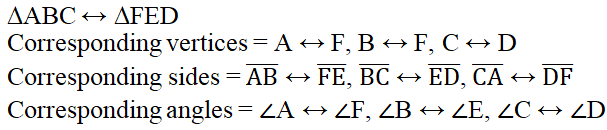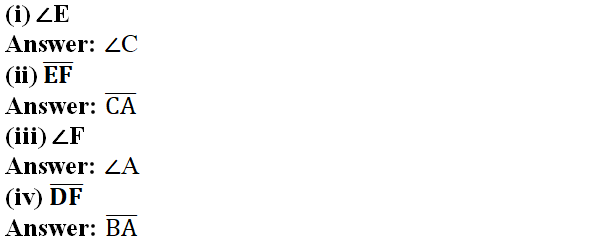# vs.eyeandcontacts.com

## Chapter 7 Congruence of Triangles Exercise 7.1

Question 1: Complete the following statements:
(a) Two line segments are congruent if they have the same length.
(b) Among two congruent angles, one has a measure of 70°; the measure of the other angle is
70°.
(c) When we write ∠A = ∠B, we actually mean
m ∠A = m ∠B.

Question 2: Give any two real-life examples for congruent shapes.
Two real-life examples for congruent shapes are -
1. Size of chocolate in the same brand.
2. Size of pens in the same brand.

Question 3: If ΔABC ≅ ΔFED under the correspondence ABC ↔ FED, write all the corresponding congruent parts of the triangles.Question 4: If ΔDEF ≅ ΔBCA, write the part(s) of ΔBCA that correspond to